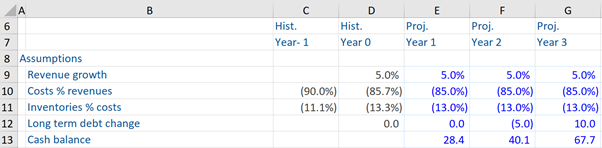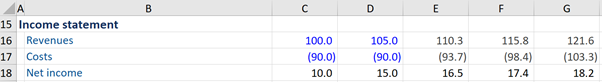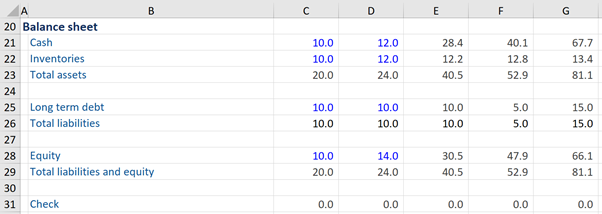## What is “Financial Forecasting”?

Financial forecasting involves taking a company’s historical financial data and using it to build future output estimates. It has a fundamental structure, which includes four main areas plus a logical process linking these pieces. The four components are:

• Input historical data
• Building ratios and statistics based on the historical data
• Making future assumptions
• Forecasting financial data

All financial models should have the same key building blocks. Regardless of how simple or detailed the model is.

## Key Learning Points

• The starting point of financial forecasting is always historical data – which is usually publicly available or can be obtained privately from the management of the company
• The modeler has several design choices to make when constructing a forecast model
• There are 4 parts to financial forecasting which are all linked together
• For financial forecasting, we need to know how the three financial statements integrate together

## Financial Forecasting – The Four Components

1. Historical data: The starting point for financial forecasting is always the historical data (publicly available or can be obtained from private sources such as company management). It is used to understand the business drivers, to form the basis for forecast assumptions, and to sense check the forecast against the history.

2. Building ratios and statistics (based on the historical data): once historical data has been inputted into a model, an analyst can look for patterns and trends in the data. This may be year-over-year percentage changes or comparing ratios such as sales/profit. Two years of historic sales may show 5% growth per annum and this would be a useful statistic for looking at future sales expectations. Analysts should look through all the data for key links and performance drivers. Ratios vary from sector to sector and an understanding of the key links and relationships between numbers is essential for producing a reasonable forecast. These ratios will also be used as a benchmark for the forward assumptions.

3. Making future assumptions (the second component above links very nicely with the third component): assuming we have witnessed that sales have increased historically by 5% every period, we may assume that future sales might also increase by 5% per annum. Historical data is the primary place to look for assumptions but there may also be some important influence and inputs from industry research (such as expanding demand for products or markets).

4. Forecasting financial data: the final financial forecasting step is to use the forward assumptions to build the forecast financial data. For example, we can now calculate that if last year’s sales were \$100, and we are assuming sales will grow by 5%, then \$100 x 1.05 = \$105 sales expectation for next year.

## Designs of Financial Models

Having stated the above, the modeler has many design choices to make when constructing an excel model. This usually depends on the size of the data being analyzed. The model design will help decide where to put the data (how many sheets to use and whether the income statement is on a different tab to the cash flow data) and where to put the ratios and model assumptions (i.e. treatment of the forward assumptions which drive the model output). Models can also vary in how to format negative numbers and other features.

Where to Put the Assumptions

The first design choice is where to put assumptions – are they kept within the financial data or have they put in a segment together in a ‘block’? The forward assumptions usually contain a wide breadth of data points ranging from sales assumptions to cost forecasts plus cash flow and balance sheet data. They can be based on year-on-year changes (such as sales and COGS growth) or on ratios (such as calculating tax as a % of pre-tax profit). Some analysts find it helpful to keep this ‘hard-coded’ data as a block. Alternatively, some modelers prefer to have the assumptions spread throughout the output or model so they are near the output cells. Blocked assumptions are particularly useful in larger models – keeping them all in one tab.

Using Positive or Negative Costs

The next design choice is whether to have costs (such as operating costs and tax expense) shown as a positive or negative figure. The advantage of showing them as negatives makes it easier to sense-check when printed (and they can be easily used with the SUM function in Excel). It is important to use the same format throughout the model so it is easy to read. Analysts have to be careful with negative figures to make sure they are correctly used for ratios. For example, tax rates can be calculated from a negative expense figure but shown as positive percentages and it must remain consistent through the model.

One Tab or Multi-Tab Models

The last design choice is whether our model is going to be a one-tab model or a multi-tab model. A one-tab model has all the major items – income statement, balance sheet, and cash flow statements all on the same tab, and even assumptions and calculations all on one tab. This is useful for smaller models and makes importing links to new files easier. A DCF model is a good example of where we often see a single-tab model. In a multi-tab model, we have a separate tab for each – income statement, balance sheet and cash flow statement. This can work well for bigger models but it is worth noting that cross sheet (and cross tab) links use more memory and can make the spreadsheet slower to use. Leveraged Finance models are often in multi-tab format.

## Financial Forecasting – Relationship between the Income Statement, Balance Sheet Statement, and Cash Flow Statement

In financial modeling, we need to know exactly how the three financial statements integrate together. For example, the cash flow statement gives a lot of detail that can help to explain the changes in cash flows within the balance sheet. Further, a balance sheet is not just made up of assets. It is also made up of liabilities and equity too. We can find more detail on equity via another financial statement – the income statement. So when the three financial statements come together, it can give us a broad view of how a particular business is doing.

## Financial Forecast Model – Example

Given below is an example of how to build an abridged simple forecast model.

Here in table 1, we can build the income statement and the balance sheet assumptions for the projected (or forecast) period. To start, we are provided with some historical data in the income statement, which can be inputted to build some ratios and statistics. These are instrumental in deciding the future forecast assumptions such as sales growth, and projected inventories as a percentage of sales.

We use the assumptions to calculate forecast data. An important first step is to look at the historical data and sum up revenues and costs to obtain historical data on net income which wasn’t provided as a standalone figure. We can do the same with the balance sheet too (i.e. sum up total assets, total liabilities, and total liabilities and equity). Then we perform the check on the balance sheet to make sure total assets equal total liabilities (and the balance sheet ‘balances’ – see Table 3). Thereafter, we can copy these formulas into period 1 of the projected period.

In going through the model below, we first calculate period 1 in full, then we copy all of the formulas for period 1 to the right into period 2 and period 3.

Looking at the example in more detail, we use the assumption of 5% revenue growth for period 1, based on last year’s revenue growth and can use this to drive a projected sales growth figure for period 1. We perform a similar procedure for costs – basing assumptions on historical data and then building up forecasts. From this process, we can obtain the net income forecast for period 1.

The next step is to carry out a similar procedure for the balance sheet (looking up assumptions and multiplying by the required variable) to obtain the cash and inventory forecast for period 1. Thereafter, we obtain the total assets (adding these forecasts up). Analysts can do the same for long-term debt and other balance sheet details.

As far as equity is concerned, we add to the net income forecast for period 1 (i.e. 16.5) and add it to last year’s equity (14.0) to get the equity for period 1(30.5). Next, we check that the balance sheet balances (Check = 0).

So following these steps we have completed period 1 forecasting in our simple model. Now we can copy the formulas to the right to get forecasts for future years. This process should grab all the assumptions from period 1 to bring them into the period 2 forecast period to get forecast figures for period 2. This process can be used for the total forecasting period.

Finally, we see that the balance sheet also balances for periods 2 and 3 (i.e. check = 0) which suggests that all the assumptions are in place and feeding through the financial statements.

Table 1 – Creating assumptionsTable 2 – Building forecastsTable 3 – Linking up the Balance Sheet## Remember to sense-check forecasts

It is important in financial modeling to maintain consistency both with assumptions rationale and when writing formulas. Analysts must also keep ‘sense-checking’ forecast outputs to make sure they remain sensible and consistent with the market conditions along with other factors. It is useful to look at the margins created from forecasted figures to see how they compare with industry averages and the historical performance of the company.

In our model, we assume sales will grow at 5% p.a. and costs will remain 85% of total revenues. This may become unrealistic over the long term as the company may see benefits from larger purchasing of raw materials or better prices from suppliers. Perhaps by sense checking this, we can decide whether we are likely to see operating margins improve and can build this into the longer-term forecasts by slightly lowering the costs as a % of revenues. Alternatively, there may be rising costs as a % of revenues due to a sustained rise in oil pushing up the price of raw materials.

Take our online research analyst course and learn how to build robust financial forecasts and error-free models critical for investment decisions.